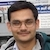# NAEST 2016 Screening Test: Frictional Force on Fridge MagnetBy

In screening round of National Anveshika Experimental Skills Test ( NAEST 2016 ), a question was asked on the frictional force acting on a magnet sticked to an Almirah. It is a common practice to use magnets to stick objects on the fridge or Almirah. The walls of the fridge are made up of magnetic material. The magnetic attraction and frictional force between the magnet and the wall help in sticking.

## Question from NAEST 2016

The mass of magnet is $m$ and the mass of Almirah is $M$. The coefficient of friction between the magnet and the Almirah is $\mu$. Choose the correct option(s) from the following:

1. Frictional force on the magnet is $f=mg$
2. Frictional force on the magnet is $f=\mu mg$
3. Frictional force on the Almira is $f=\mu Mg$
4. Normal force between the magnet and Almirah is $N=mg/\mu$

Solution: The forces acting on the magnet are

1. gravitational force $mg$ (downwards)
2. frictional force $f$ (upwards)
3. magnetic force $F_B$ (into the wall)
4. normal reaction $N$ (out of the wall)The net force on the magnet is zero because it is in equilibrium. Equate forces in the horizontal and the vertical directions to zero to get \begin{align} f&=mg,\\ F_B&=N. \end{align} Thus, frictional force on the magnet is equal to its weight i.e., $f=mg$.

The limiting value of frictional force is \begin{align} f_\text{max}=\mu N=\mu F_B \end{align} The magnet will fall down if its weight is more than $\mu F_B$. Thus, a magnet gets stuck to the wall if its mass is small, magnetic strength is large, and the coefficient of friction is large. The minimum value of magnetic force required to stick a magnet is \begin{align} F_\text{B, min}=mg/\mu. \end{align} If the magnetic force is less than $mg/\mu$ then the magnet will fall down. You cannot stick a weak magnet.